Today, you can produce a wide range of choice model experimental designs with numerous different algorithms. But with all this design diversity, how do you measure its quality? In this post, I'll show you how you can distill your data into a few key diagnostic metrics of balance, which will help you assess the quality of your choice model design.

## Defining Balance and Overlap

Often, the quality of a design is described in terms of its balance and overlap. Balance is a measure of consistency of the frequencies of the attribute levels. Overlap is a measure of repetition of attribute levels within the same question.

However, the drawback of these measures is that they produce many statistics that are difficult to interpret in isolation. In order to develop an understanding of how good your design is, you must look at these statistics as part of the bigger picture. I'll show you how to derive diagnostic metrics that provide a holistic measure of the quality of your design.

You can easily apply these metrics to compare designs created from different algorithms.

## An example design

In Q or Displayr designs are created with Conjoint/Choice Modeling > Experimental Design. I am using a small design produced with the Random algorithm. There are two attributes (Color and Speed), each of which have three levels. Every respondent answers five questions, each of which contains three alternatives. There are two versions.

Below I show the output of Conjoint/Choice Modeling > Diagnostic > Experimental Design > Balances and Overlaps. Don't worry if you're confused about what each output means. I'm about to explain them.

D-error and Overlaps

D-error is a measure that shows how good or bad a design is at extracting information from respondents. A lower D-error indicates a better design. Usually, D-errors are used to compare the quality of designs created by different algorithms.

For each attribute, the overlap is calculated as the percentage of questions with some repetition of a level. The number of levels of each attribute are shown in brackets. In the example above, 70% of the questions have at least one repeated Color level. In other words, 30% of questions show alternatives with distinct Color levels.

## Balance statistics

In order to take all our complex data and distill it into a few metrics we can easily use to see how good a design is, we're going to have to do some calculations.

To calculate the balance of an attribute within a version, first define the mean level frequency for attribute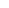as,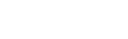where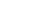is the number of questions per respondent,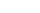is the number of alternatives per question and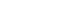is the number of levels of this attribute. Since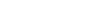is the total number of appearances of each attribute in the version,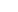is the number of times each level appears if the levels are balanced.

The balance of an attribute is then defined as the sum across levels of the absolute differences between the level frequency and mean level frequency.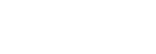where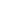is the frequency of occurrence of levelfor attributein the design version.

To normalize the balance, define the worst possible balance of the attribute as,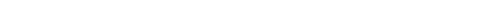The first term in brackets arises from one level appearing in all alternatives. The second term in brackets arises from all other levels never appearing.

The normalized balance for this version and attribute is calculated according to,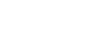To calculate the mean version balance, take the average of the normalized balances across all attributes and all versions. If a version is perfectly balanced and the levels of each attribute appear the same number of times, mean version balance is one.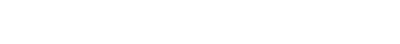By calculating the balance for the whole design regardless of version, I arrive at the analogous across version balance. If this value is one, the levels of each attribute appear the same number of times within the whole design. Note that the across version balance could be one despite the individual versions not being balanced (so across version balance is less than one). The more usual case is that across version balance is closer to one because at the whole-design level, some of the individual version differences are offsetting.

## Worked example of balance

Using the example shown previously,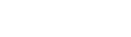With frequencies of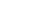in the first version as follows,

The calculations for the balance, worst balance and normalized balance ofare,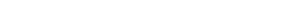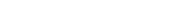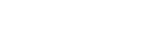For all attributes and versions the table of of normlized balances is,

Taking the average arrives at mean version balance of 0.825 as above.

In the original diagnostic output the singles lists are the level balances across the whole design. These can be used to calculate the across version balance. Without going through each step,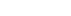,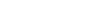and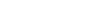. Since those values are the same for the Speed attribute, across version balance = 0.9.

## Pairwise balances

It is relatively straightforward for an algorithm to maintain single level balance (apart from for the Random algorithm!). More challenging is the pairwise balance. I discuss why you want your designs to be pairwise balanced here.

The pairwise balance of two attributes is best shown by a table of the co-occurrences of each pair of levels. Below I reproduce the pairs table from the diagnostic output. Using the bottom right cell as an example, it shows that across the whole design there were 3 alternatives that were both Yellow and Slow.

The formulae for balance statistics can be converted to pairwise balance statistics (where and b are attributes) as follows,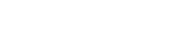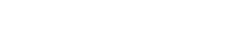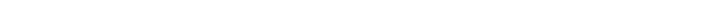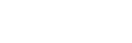To calculate the mean version pairwise balance, take the average of the normalized balances across all distinct pairs of attributes and all versions.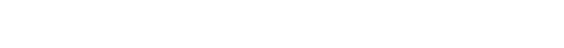Like the mean version balance, if mean version pairwise balance is one, then each pair of levels for each pair of attributes occurs equally often in each version. The closer that mean version pairwise balance is to zero, the more imbalanced the design. Across version pairwise balance is the counterpart of across version balance. It ignores versions and considers the pairwise balance of the design as a whole.

## Worked example of pairwise balance

The table of pairwise frequencies for the first version is as follows,

For which we can compute the statistics according to the above formulae.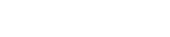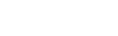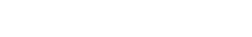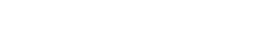For the second version,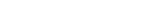which gives the mean version pairwise balance of 0.6625. Remember that the closer the mean version pairwise balance is to zero, the more imbalanced the design is.

## Conclusion

I hope this helps you distill your data into a few metrics that give you a better idea of the quality of your design. You can easily calculate these statistics in Q, Displayr or R. If you would like to see my worked example and adapt it for your own data, you can in this Displayr document.

The power of these metrics is in using them as benchmarks for comparison between different designs. In a later post I will use this technique to explore the differences between design algorithms.

Find out more about Choice Model Experimental Designs on our Displayr blog. Want to do this yourself? Try Displayr for free. We've specifically designed, what we think, is the best choice modeling software in the world.